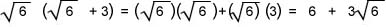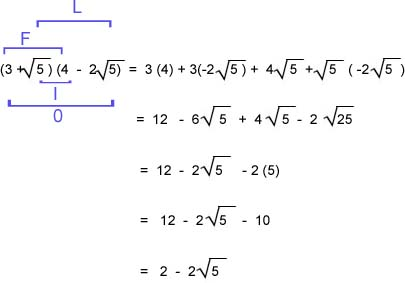Multiply Square Roots

Multiply Square Roots

The distributive property can be used to multiply radical expressions.

ExampleTo multiply two binomials with square root terms, each term in the first binomial must be multiplied by each term in the second binomial.  This can be accomplished using the FOIL method.

F  = First terms in each binomial

O = Terms on the outside of each binomial

I  = Terms on the inside of each binomial

L = Last terms in each binomial

Example